A domain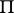of the complex space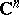,, which can be represented by inequalities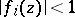, where the functions,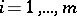, are holomorphic in some domaincontaining, i.e.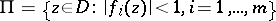. It is also assumed thatis compact in. If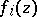are polynomials, the analytic polyhedron is said to be a polynomial polyhedron. If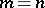and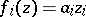, the analytic polyhedron is called a polydisc. The sets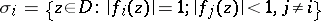are called the faces of the analytic polyhedron. The intersection of anydifferent facesis said to be an edge of the analytic polyhedron. Ifand all faces have dimension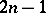, while no edge has dimension exceeding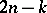, the analytic polyhedron is a Weil domain. The set of-dimensional edges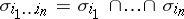forms the skeleton of the analytic polyhedron. The concept of an analytic polyhedron is important in problems of integral representations of analytic functions of several variables.